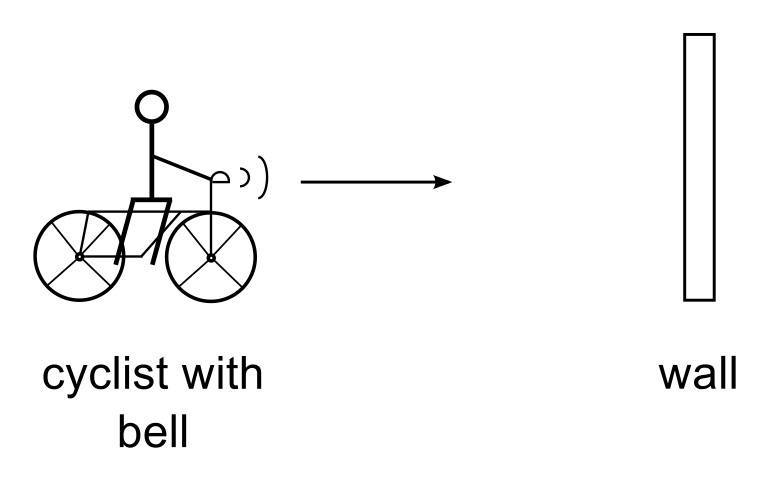# Doppler effect and concept of Beat Problem

• MMONISM

## Homework Statement

A cyclist with a bell ringing with a frequency of 658.8 Hz drives towards a wall with a speed of 3.18 ms-1. Just before colliding with the wall the cyclist hears beats, due to the bell itself and the reflection of the sound from the wall. What is the frequency of beats detected by the observer? Assume that the velocity of sound in air is 343 ms-1.## Homework Equations

f'=f(c+v0)/(c-vs)
fbeat = f1 - f2

## The Attempt at a Solution

f'=f(c+v0)/(c-vs)=f(c)/(c-3.18 )=665 Hz
and I am stuck here, I used fbeat = 665 - 658.8 = 6.2 Hz which is incorrect. Could someone help me please?

Last edited:

## Homework Statement

A cyclist with a bell ringing with a frequency of 658.8 Hz drives towards a wall with a speed of 3.18 ms-1. Just before colliding with the wall the cyclist hears beats, due to the bell itself and the reflection of the sound from the wall. What is the frequency of beats detected by the observer? Assume that the velocity of sound in air is 343 ms-1.View attachment 84371

## Homework Equations

f'=f(c+v0)/(c-vs)
fbeat = f1 - f2

## The Attempt at a Solution

f'=f(c+v0)/(c-vs)=f(c)/(c-3.18 )=665 Hz
and I am stuck here, I used fbeat = 665 - 658.8 = 6.2 Hz which is incorrect. Could someone help me please?
The wall "observes" sound from the cyclist, a moving source, which is at frequency higher than the original. It reflects sound with the modified frequency, and it is heard by the cyclist, as moving observer.

•MMONISM
The wall "observes" sound from the cyclist, a moving source, which is at frequency higher than the original. It reflects sound with the modified frequency, and it is heard by the cyclist, as moving observer.
Ok, so f'=f(c+v0)/(c-vs)=f(c)/(c-3.18 )=665 Hz
f''=f'(c+3.18)/(c)=671.2Hz
fbeat = 671.2 - 658.8 = 12.4 Hz
Is this correct now?

I think it is right.

•MMONISM